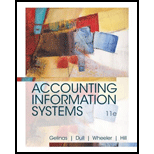Chapter 6, Problem 2SPAccounting Information Systems

11th Edition
Ulric J. Gelinas + 3 others
ISBN: 9781337552127

Solutions

Chapter
SectionAccounting Information Systems

11th Edition
Ulric J. Gelinas + 3 others
ISBN: 9781337552127
Textbook Problem

Develop the REA data model from Short Problem 6-1 into an E-R diagram with maximum cardinalities. Assume that each inventory item can be ordered multiple times. Draw the model using Microsoft Visio (or other software).Note: Short Problems 6-3 and 6-4 should be completed with a database software package such as Access.

Summary Introduction

To develop: REA data model for ordering inventory into an E-R diagram with maximum cardinalities.

Explanation

Resources-Events-Agents (REA) model:

• This model tells how the accounting system can be re-engineered for the computer age. This model helps in eliminating many accounting objects that are not that necessary in the computer age.

Justification:

• Resources-Events-Agents (REA) model ought to be drawn into an E-R diagram with maximum cardinalities assuming that each inventory item can be ordered multiple times.
• Models are the simplified versions of an entity. It also helps in identifying and understanding the functional aspects of as entity...

Still sussing out bartleby?

Check out a sample textbook solution.

See a sample solution

The Solution to Your Study Problems

Bartleby provides explanations to thousands of textbook problems written by our experts, many with advanced degrees!

Get Started

Find more solutions based on key concepts